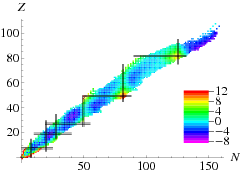$$\require{cancel}$$

# 4.4: Nuclear mass formula

•• Contributed by Niels Walet
• Professor (Physics) at University of Manchester

There is more structure in Figure 4.3.1 than just a simple linear dependence on $$A$$. A naive analysis suggests that the following terms should play a role:

1. Bulk energy: This is the term studied above, and saturation implies that the energy is proportional to $$B_{\text{bulk}}=\alpha A$$.
2. Surface energy: Nucleons at the surface of the nuclear sphere have less neighbors, and should feel less attraction. Since the surface area goes with $$R^2$$, we find $$B_{\text{surface}}=-\beta A$$.
3. Pauli or symmetry energy: nucleons are fermions (will be discussed later). That means that they cannot occupy the same states, thus reducing the binding. This is found to be proportional to $$B_{\text{symm}}=-\gamma (N/2-Z/2)^2/A^2$$.
4. Coulomb energy: protons are charges and they repel. The average distance between is related to the radius of the nucleus, the number of interaction is roughly $$Z^2$$ (or $$Z(Z-1)$$). We have to include the term $$B_{\text{Coul}}=-\epsilon Z^2/A$$.Illustration of the terms of the semi-empirical mass formula in the liquid drop model of the atomic nucleus. Image used with permission (CC BY-SA; Daniel FR).

Taking all this together we fit the formula

$B(A,Z) = \alpha A - \beta A^{2/3} - \gamma (A/2-Z)^2A^{-1} - \epsilon Z^2 A^{-1/3} \label{eq:mass1}$

to all know nuclear binding energies with $$A\geq 16$$ (the formula is not so good for light nuclei). The fit results are given in Table $$\PageIndex{1}$$.

Table $$\PageIndex{1}$$: Fit of masses to Equation \ref{eq:mass1}.
parameter value
$$\alpha$$ 15.36 MeV
$$\beta$$ 16.32 MeV
$$\gamma$$ 90.45 MeV
$$\epsilon$$ 0.6928 MeVFigure $$\PageIndex{1}$$: Difference between fitted binding energies and experimental values (color), as a function of $$N$$ and $$Z$$.

In Table $$\PageIndex{2}$$ we show how well this fit works. There remains a certain amount of structure, see below, as well as a strong difference between neighbouring nuclei. This is due to the superfluid nature of nuclear material: nucleons of opposite momenta tend to anti-align their spins, thus gaining energy. The solution is to add a pairing term to the binding energy,

$B_{\text{pair}} = \begin{cases} A^{-1/2} & \text{for N odd, Z odd}\\ - A^{-1/2} & \text{for N even, Z even}\end{cases}$

The results including this term are significantly better, even though all other parameters remain at the same position, see Table $$\PageIndex{2}$$. Taking all this together we fit the formula

$B(A,Z) = \alpha A - \beta A^{2/3} - \gamma (A/2-Z)^2A^{-1} - \delta B_{\text{pair}}(A,Z)-\epsilon Z^2 A^{-1/3} \label{eq:mass2}$

Table $$\PageIndex{2}$$: Fit of masses to Equation \ref{eq:mass2}.
parameter value
$$\alpha$$ 15.36 MeV
$$\beta$$ 16.32 MeV
$$\gamma$$ 90.46 MeV
$$\delta$$ 11.32 MeV
$$\epsilon$$ 0.6929 MeVTable $$\PageIndex{3}$$: $$B/A$$ versus $$A$$, mass formula subtracted